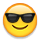# FIRSTNONBLANK() as a FunctionAdd a "FIRSTNONBLANK()" function that will evaluate each of the arguments and return the first one that is not blank.

This would allow for formulas like the following to be greatly simplified and potentially better performing:

Example 1:
IF ISNOTBLANK( line item A) THEN line item A

ELSE IF ISNOTBLANK( line itemTHEN line item B

ELSE IF ISNOTBLANK( line item C) THEN line item C

ELSE default value

Alternative with a FIRSTNONBLANK() function:
FIRSTNONBLANK( line item A, line item B, line item C, default value)

In the case above, the performance difference is probably not that much, but it is much simpler.   But here is an example where the the performance difference could be significant

IF ISNOTBLANK(FINDITEM( list, code 1)) THEN FINDITEM( list, code 1)
ELSE IF ISNOTBLANK(FINDITEM( list, code 2)) THEN FINDITEM( list, code 2)

ELSE IF ISNOTBLANK(FINDITEM( list, code 3)) THEN FINDITEM( list, code 3)
ELSE BLANK

Here the FINDITEM is potentially being done twice when it is found.  This formula can be greatly simplified and improved from a performance standpoint if there were a FIRSTNONBLANK() function:

FIRSTNONBLANK(FINDITEM( list, code 1), FINDITEM( list, code 2), FINDITEM( list, code 3))

Tagged:
8